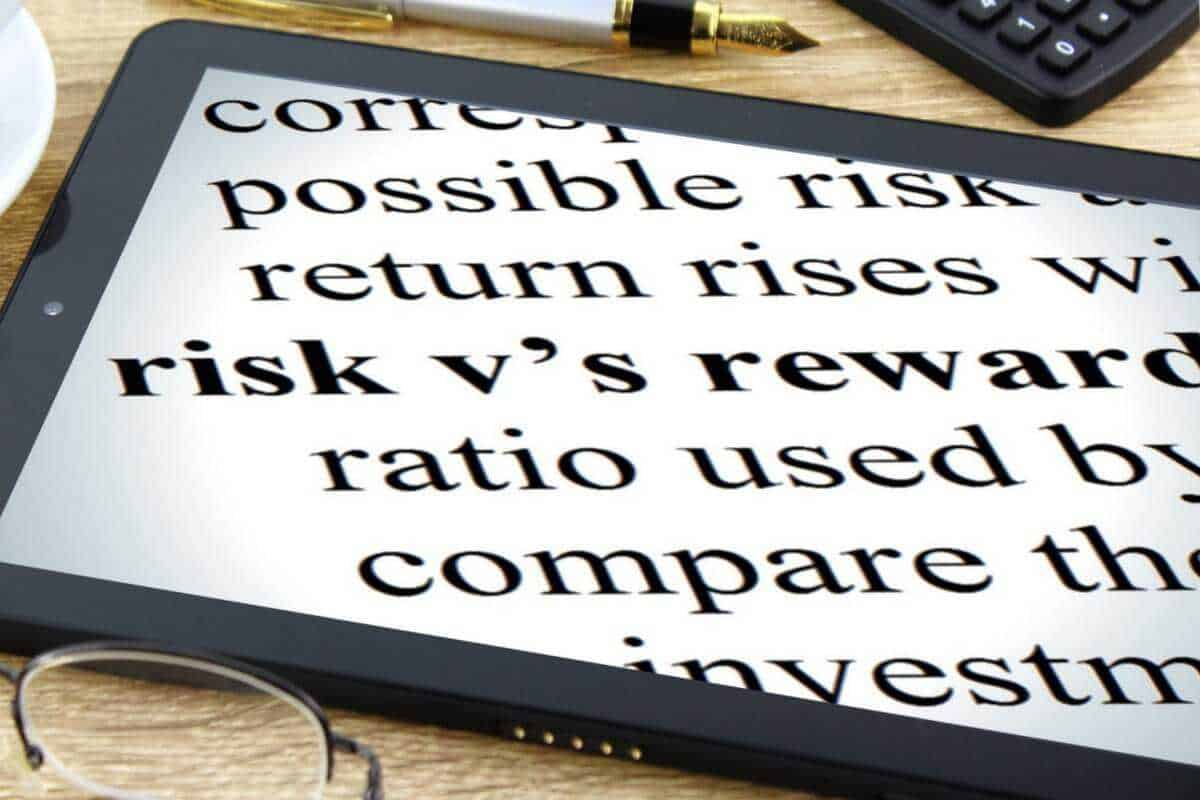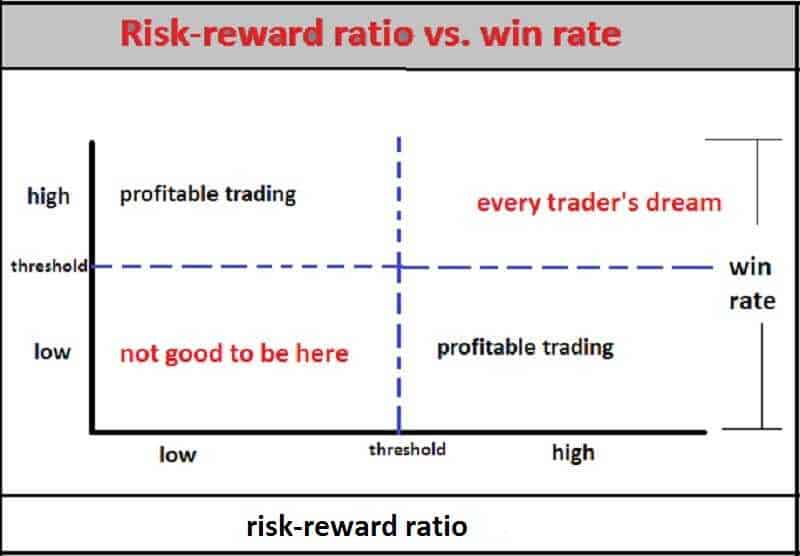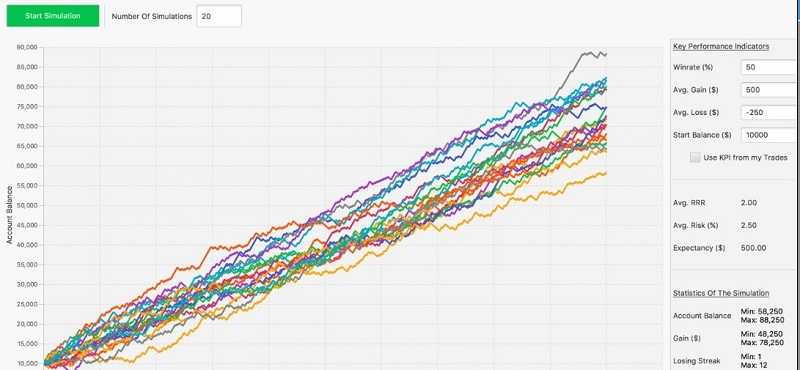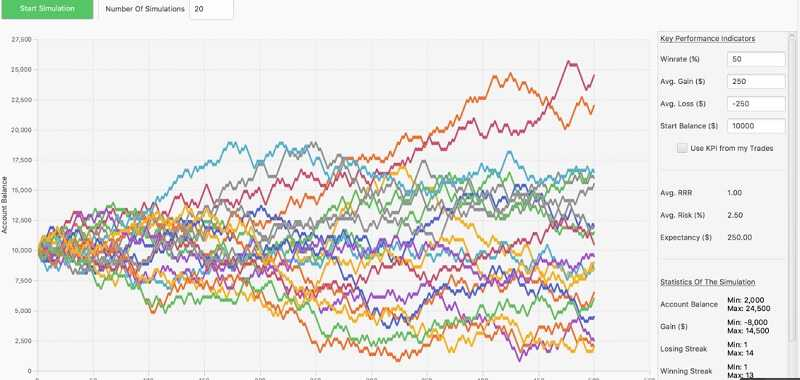## How to use the risk-reward ratio efficiently in trading

The risk-reward ratio is a formula used to measure the expected gains of a given investment against the risk of loss.

It is typically shown as a figure for the assessed risk separated by a colon from the figure for the prospective reward. The adequate ratio can vary, but trade experts recommend a ratio between 2:1 and 3:1 to determine a worthy investment.

Typically, the ratio quantifies the relationship between the potential money lost, should the investment or action fail, versus the money realized if all goes as planned.

In other words, the risk-reward ratio measures how much your potential reward is, for every coin you risk.

For example:

If you have a risk-reward ratio of 1:2, it means you’re risking \$1 to potentially make \$2.

But, if you have a risk-reward ratio of 1:4, it means you’re risking \$1 to potentially make \$4.
Investors use the risk-reward ratio to define the viability or worthiness of a given investment. You can limit risk by issuing stop-loss orders,  sometimes. That will trigger automatic sales of stock or other securities when they hit a particular price. If you don’t implement such a mechanism in place, the risk is likely endless.

### How to use the risk-reward ratio like a pro

Let’s be clear: the win rate in trading it totally trivial on its own. Some traders put way too much importance on the win rate. At the same time, they don’t understand that a win rate will not tell you anything about the quality of a practice or a trader.

The truth is that trader can lose money with a 70% or even with a 90% win rate if few losers are so big that they wipe out trader’s winners. But, the trader can have a successful system even with a win rate of 40% or only 30% if such a trader lets winners run and cut losses short.

It all comes down to the reward-risk ratio.

The reward to risk ratio (RRR, or reward risk ratio) is probably the most important metric in trading. A trader who understands the RRR can improve his chances of becoming profitable.

### Myth 1

“You need a minimum of 1:2 risk reward ratio.”

That is nonsense.

Why?

Because the risk-reward ratio is insignificant on its own.

We will give you an example:

Let’s say you have a risk-reward ratio of 1:2. This means, for every trade you win, you make \$2.

But, your winning rate is 30%.

To have a clear picture, out of 10 trades, you have 7 losing trades and 3 winners.

Let’s do the math…
Total Loss = \$1 * 7 = -\$7
Total Gain = \$2 * 3 = \$6
Net loss = -\$1

You see, you are not the winner.

And you understand the risk-reward ratio by itself is an insignificant metric.

There is nothing like good or bad risk-reward ratios. It just comes down to how you use it. You can even trade profitably with a risk-reward ratio of 1:1 or less.

So, you must combine your risk-reward ratio with your winning rate to know whether you’ll make money in the long run. That is known as your expectancy.

### The risk-reward ratio is useless

Some traders like to say the reward-risk ratio is useless. It cannot be further from the truth.

When you use the RRR in combination with other trading metrics, such as win rate, for example, it instantly matures as one of the most important trading tools.

Without knowing the risk-reward ratio of a single trade, it is literally impossible to trade profitably.

##### The risk-reward ratio isn’t enough

Do you want to know the secret code?

Here it is.
E= [1+ (W/L)] x P – 1

Now let’s put this all together and let’s take a look at some performance statistics and how the RRR fits in.

Below, we see a performance simulation based off a strategy with a win rate of 50% and a risk of 2.5% per trade. The RRR was first set to 2:1 on average per trade.You can see that out of those 20 simulated outcomes (the different graphs), all of them were positive after 500 trades.

Remember, with a win rate of 50%, you just need a RRR greater than 1:1 to trade profitably. With a 2:1 RRR you can potentially trader very profitable with a win rate of 50%.

Now let’s take a look when the only thing we change is the RRR. Everything else is the same.

Assume that each trade has a RRR of 1:1.

Out of the 20 simulated outcomes, only a few will generate a positive outcome and many show a negative outcome.

To conclude, with a win rate of 50%, trading a RRR of 1:1 is very volatile and variance will be huge. You have to remember, with a win rate of 50%, you need a RRR greater than 1:1.

Now let’s take a look when the only thing we change is the RRR. Everything else is the same.

Assume that each trade has a RRR of 1:1.

Out of the 20 simulated outcomes, only a few will generate a positive outcome and many show a negative outcome.To conclude, with a win rate of 50%, trading a RRR of 1:1 is very volatile and variance will be huge. You have to remember, with a win rate of 50%, you need a RRR greater than 1:1.

#### The bottom line

Portfolio with more unknown factors may have a higher probability of failure but at the same time offer a significantly higher return if they are successful. The ideal is the low risk-reward ratio – the little risk of failure and a high potential for reward.

risk disclosure

#### When unsure what's the right move, you can always trade Forex

##### Get the number #1 winning technical analysis strategy for trading Forex to your email.
Containing the full system rules and unique cash-making strategies. You'll be surprised to see what indicators are being used and what is the master tuning for successful trades. Including case-studies and images.